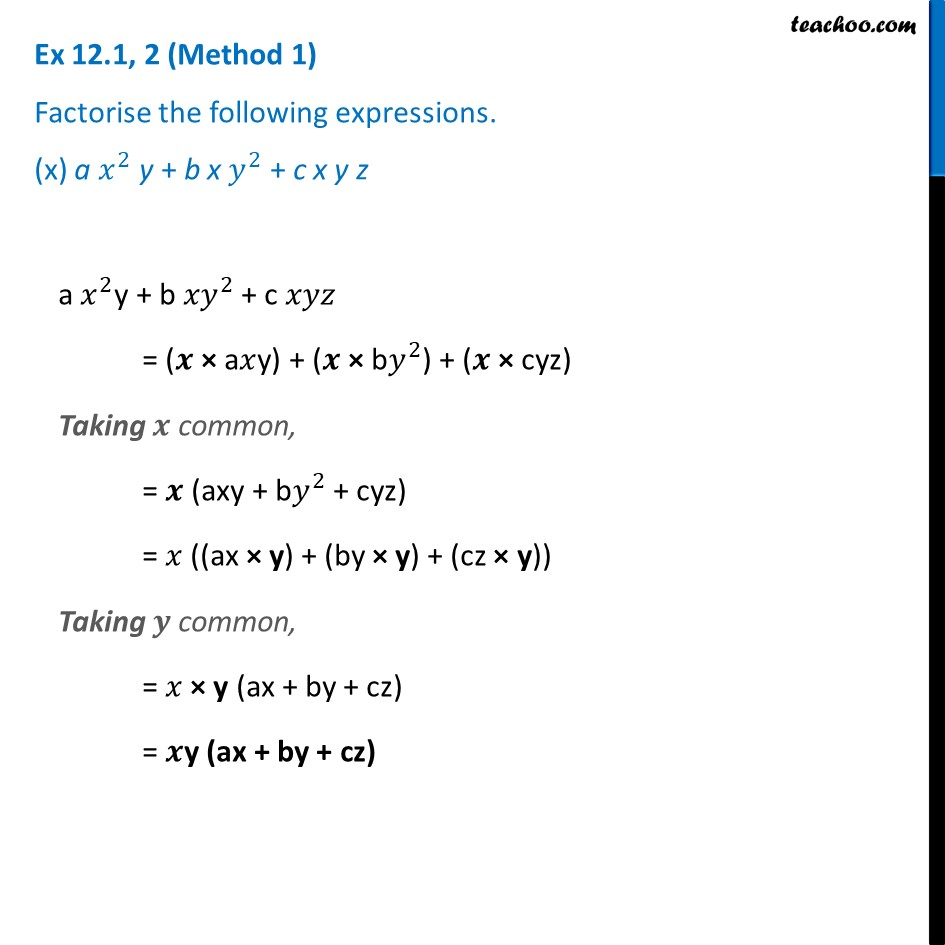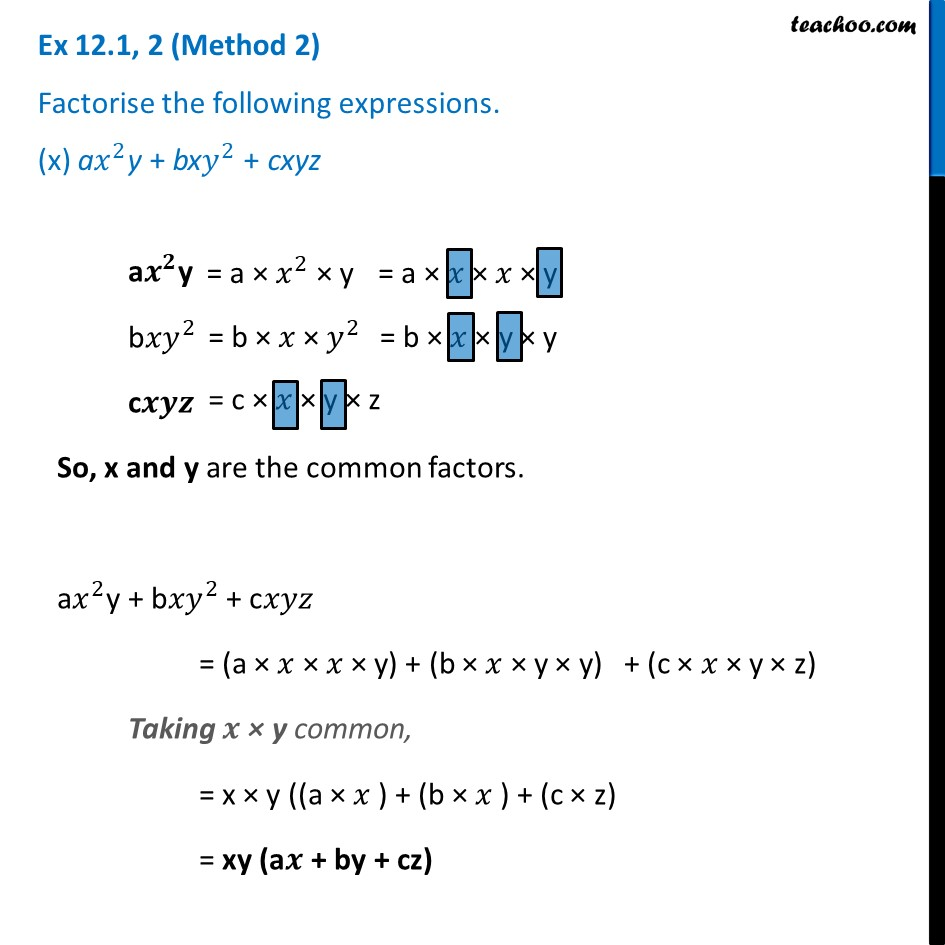Ex 12.1

Chapter 12 Class 8 Factorisation
Serial order wiseLearn in your speed, with individual attention - Teachoo Maths 1-on-1 Class

### Transcript

Ex 12.1, 2 (Method 1) Factorise the following expressions. (x) a 𝑥^2 y + b x 𝑦^2 + c x y za 𝑥^2y + b 〖𝑥𝑦〗^2 + c 𝑥𝑦𝑧 = (𝒙 × a𝑥y) + (𝒙 × b𝑦^2) + (𝒙 × cyz) Taking 𝒙 common, = 𝒙 (axy + b𝑦^2 + cyz) = 𝑥 ((ax × y) + (by × y) + (cz × y)) Taking 𝒚 common, = 𝑥 × y (ax + by + cz) = 𝒙y (ax + by + cz) Ex 12.1, 2 (Method 2) Factorise the following expressions. (x) a𝑥^2y + bx𝑦^2 + cxyz a𝒙^𝟐y b〖𝑥𝑦〗^2 c𝒙𝒚𝒛 So, x and y are the common factors. a𝑥^2y + b〖𝑥𝑦〗^2 + c𝑥𝑦𝑧 = (a × 𝑥 × 𝑥 × y) + (b × 𝑥 × y × y) + (c × 𝑥 × y × z) Taking 𝒙 × y common, = x × y ((a × 𝑥 ) + (b × 𝑥 ) + (c × z) = xy (a𝒙 + by + cz) = a × 𝑥^2 × y = b × 𝑥 × 𝑦^2 = c × 𝑥 × y × z = a × 𝑥 × 𝑥 × y = b × 𝑥 × y × y